## The quantization of space

Quantum mechanics defines our observable physical environment only in terms of the probabilistic values associated with Schrödinger’s wave equation.

More specifically it defines a particle in terms of the instantaneous collapse of a wave function which it assumes extends form one edge of the universe to the other.

However this definition appears to contradict two very basic properties of our observable reality: the fact that particles appear to have a physical presents and how it can be simultaneously be in many places at the same time.  In other words if Schrödinger’s wave equation does define our observable environment one should be able to explain how a mathematical probability obtains a physical presents when observed and how that physical presents can exist in different places at the same time.

Einstein gave us the answer to the second question in his formula for relativistic length contraction L = L0((1 – v2/c2))1/2 because it tells the distance between every point along the trajectory of all forms of energy which are moving at the speed when viewed by an outside observer including that associated with the wavefunction is zero.   Additionally because time stops for anything traveling at the speed of light it would have enough time to travel from the perspective of an outside observer from one end of the universe to the other.  In other words according to Einstein’s theory the particle associated with the wave function when viewed by an outside observer simultaneously exist in many places because the distance between its end points when viewed by him or her is zero

However it is extremely difficult to define a set of statements which explains how those probabilities can be connected to physical properties of particles even though it has held up to rigorous and thorough experimental testing.

This may be the reason most physicists consider quantum mechanics only in terms of its mathematical formalization instead trying to understand the meaning of it in terms of the space-time environment we occupy.

For example in 1924 Louis de Broglie was the first to realize all particles are physically composed of a matter wave as the discovery of electron diffraction by crystals in 1927 by Davisson and Germer) verified.  However in his paper, Theory of the double solution he could not define a physical interpretation of Schrödinger equation in classical terms of space and time.

As is pointed at his biography on the nobleprize.org web site in "1951, he together with some of his younger colleagues made another attempt, one which he abandoned in the face of the almost universal adherence of physicists to the purely probabilistic mathematical interpretation of, Bohr, and Heisenberg."

However the fact that no has been able to physically connect those probabilities to our environment does not change the fact that there must be one because if there wasn’t they could not interact with it to create the physicality of observable world upon which those probabilities are based.

As mentioned earlier Louis de Broglie and his colleagues tried unsuccessfully to find a physical interpretation of Schrödinger equation in classical terms of space and time.

However the reason for their failure may be due to the fact that quantum properties of particles are related to the spatial not a time dependent properties of the wave function.

If so one may be able to establish the connection Louis de Broglie was looking for it in terms of a spatial instead of the time or space-time property of the wave function

Einstein gave us the ability to do this when he defined the geometric properties of space-time in terms of the constant velocity of light and a dynamic balance between mass and energy because that  provided a method of converting a unit of time in a space-time environment to a unit of space in four *spatial* dimensions.  Additionally because the velocity of light is constant he also defined a one to one quantitative and qualitative correspondence between his space-time universe and one made up of four *spatial* dimensions.

The fact that one can use Einstein’s equations to qualitatively and quantitatively redefine the curvature in space-time he associated with energy in terms of four *spatial* dimensions is one bases for assuming as was done in the article “Defining energy?” Nov 27, 2007 that all forms of energy can be derived in terms of a spatial displacement in a "surface" of a three-dimensional space manifold with respect to a fourth *spatial* dimension.

This would have allowed Louis de Broglie to physically connect the probabilities associated Schrödinger equationto the observable properties of particles in terms of a physical or spatial displacement in a "surface" of a three-dimensional space manifold with respect to a fourth *spatial* dimension as was done in the article  "Why is energy/mass quantized?" Oct. 4, 2007.

Briefly that article showed that one can do this by assuming they are caused by the formation of a resonant system created by the wave component of particles on a "surface" of a three-dimensional space manifold with respect to fourth "spatial" dimension. This is because it showed the four conditions required for resonance to occur in a three-dimensional environment, an object, or substance with a natural frequency, a forcing function at the same frequency as the natural frequency, the lack of a damping frequency and the ability for the substance to oscillate spatial would occur in one made up of four.

The existence of four *spatial* dimensions would give a matter wave the ability to oscillate spatially on a "surface" between a third and fourth *spatial* dimension thereby fulfilling one of the requirements for classical resonance to occur.

These oscillations would be caused by an event such as the decay of a subatomic particle or the shifting of an electron in an atomic orbital.  This would force the "surface" of a three-dimensional space manifold with respect to a fourth *spatial* dimension to oscillate with the frequency associated with the energy of that event.

However, the oscillations caused by such an event would serve as forcing function allowing a resonant system or "structure" to be established on a surface of a three-dimensional space manifold.

Yet the classical laws of three-dimensional space tell us the energy of resonant systems can only take on the discontinuous or discreet energies associated with their fundamental or harmonic of their fundamental frequency.

However, these are the similar to the quantum mechanical properties of energy/mass in that they can only take on the discontinuous or discreet energies associated with the formula E=hv where "E" equals the energy of a particle "h" equal Planck’s constant "v" equals the frequency of its wave component.

In other words Louis de Broglie would have been able to physicality connect the quantum mechanical properties of his particle waves to Schrödinger equation in terms of the discrete incremental energies associated with a resonant system in four *spatial* dimensions if he had assume space was composed of it instead of four dimensional space-time.

Yet it also would have allowed him to define the physical boundaries of a quantum system in terms of the geometric properties of four *spatial* dimensions.

For example in classical physics, a point on the two-dimensional surface of a piece of paper is confined to that surface.  However, that surface can oscillate up or down with respect to three-dimensional space.

Similarly an object occupying a volume of three-dimensional space would be confined to it however, it could, similar to the surface of the paper oscillate “up” or “down” with respect to a fourth *spatial* dimension.

The confinement of the “upward” and “downward” oscillations of a three-dimension volume with respect to a fourth *spatial* dimension is what defines the spatial boundaries associated with a particle in the article "Why is energy/mass quantized?" Oct. 4, 2007

As mentioned earlier the article “Defining energy?” Nov 27, 2007 showed all forms of energy can be derived in terms of a spatial displacement in a "surface" of a three-dimensional space manifold with respect to a fourth *spatial* dimension.

However assuming the energy associated with Louis de Broglie particle wave is result of a displacement in four *spatial* dimension instead of four dimensional space-time as was done earlier allows one to connect the probabilities associated with Schrödinger equation to the observable properties of particles.  In other words it can explain how one can be observed at a specific point in space even though its wave energy is distributed throughout a relatively large volume of space.

For example Classical mechanics tell us that due to the continuous properties of waves the energy the article "Why is energy/mass quantized?" Oct. 4, 2007 associated with a quantum system would be distributed throughout the entire "surface" a three-dimensional space manifold with respect to a fourth *spatial* dimension.

It also tells us that the energy of a vibrating or oscillating ball on a rubber diaphragm would be disturbed over its entire surface while the magnitude of those vibrations would decrease as one move away from the focal point of the oscillations.

Similarly if the assumption that quantum properties of energy/mass are a result of vibrations or oscillations in a "surface" of three-dimensional space is correct then classical mechanics tell us that those oscillations would be distributed over the entire "surface" three-dimensional space while the magnitude of those vibrations would be greatest at the focal point of the oscillations and decreases as one moves away from it.

As mentioned earlier the article “Why is energy/mass quantized?” shown a particle is a result of a resonant structure formed on the "surface" of a three-dimensional space manifold with respect to a fourth *spatial* dimension.

Yet Classical Wave Mechanics tells us resonance would most probably occur on the surface of the rubber sheet were the magnitude of the vibrations is greatest and would diminish as one move away from that point.

Similarly a particle would most probably be observed were the magnitude of the vibrations in a "surface" of a three-dimensional space manifold is greatest and would diminish as one move away from that point.

Additionally it also gives us a classical expiation of how a particle can simultaneously exist in many different place and why the probability of finding it in a give volume of space is what it is because as mentioned earlier according to Einstein’s theory the distance between end points of the particle wave when viewed by an outside observer is zero  even though it may extend from one end of the universe to the other because it is traveling at the speed of light.    In other words according to Einstein’s theory the wave function when viewed by an outside observer simultaneously exist in many places therefore there is a probability of finding the resonant structure associated with its particle properties any where in that space.

This shows how one can connect the properties with Schrödinger’s equation to our observable environment by assuming that space is quantized by the resonate system created by a physical wave in either space-time dimension or a "surface" of a three-dimension space manifold with respect to fourth "spatial" dimension and observing it causes it to collapse into the smaller volume of a resonant structure Quantum Mechanics associates with a particle.

It should be remember Einstein’s genius allows us to choose to define a quantum system in either a space-time environment or one consisting of four *spatial* dimension when he defined the geometry of space-time in terms of the constant velocity of light. This interchangeability broadens the environment encompassed by his theories thereby giving us a new perspective on the probabilistic properties of a quantum environment and how they physically connected to our observable universe.

Later Jeff

 Anthology of The Imagineer’s Chronicles Vol. 1 thru 7 2007 thru 2016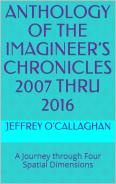Ebook \$10.50 he Reality of the Fourth Spatial DimensionPaperback \$9.77 Ebook    \$6.24 The Imagineer’s Chronicles Vol. 7 — 2016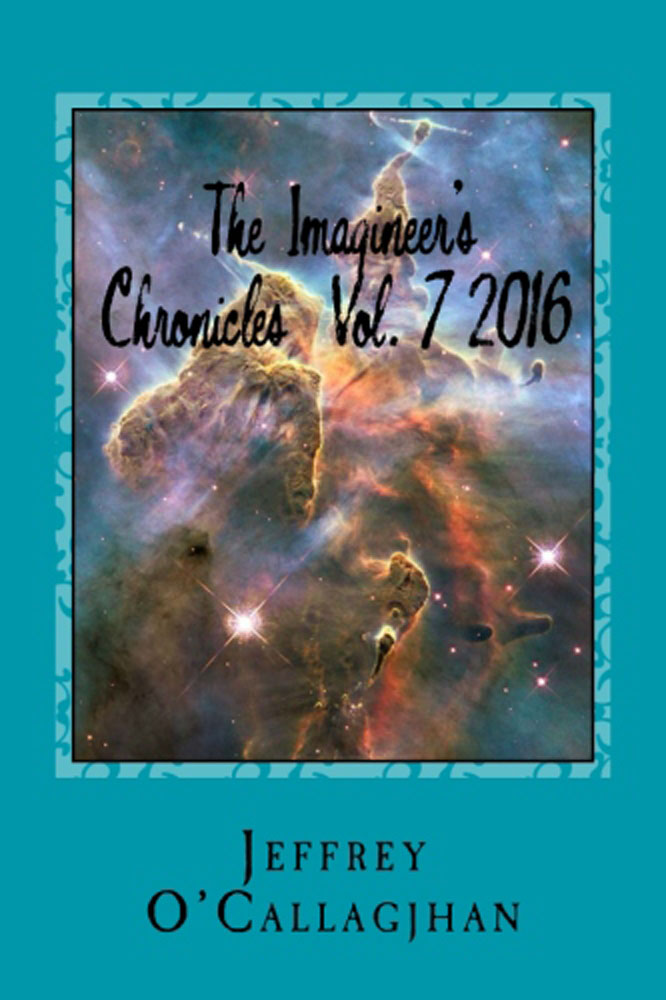Paperback \$11.25 E-book \$7.25 The Imagineer’s Chronicles Vol. 6 — 2015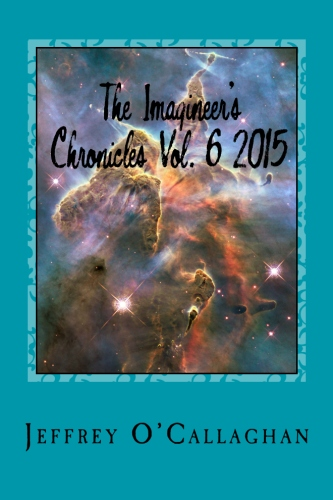Paperback \$12.25 E-book \$9.89 The Imagineer’s Chronicles Vol. 5 — 2014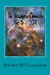Paperback \$14.84 Ebook \$9.97 The Imagineer’s Chronicles Vol. 2 — 2011Paperback \$9.24 Ebook \$6.57 The Imagineer’s Chronicles 2007 thru 2010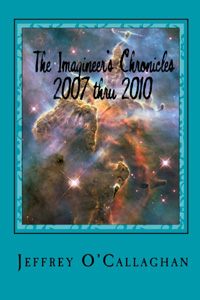Paperback \$15.43 Ebook \$7.82see more...